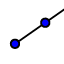# Constructing Angles: Ex. 6

Topic:
Angles

## DIRECTIONS:

Use the RAY, ANGLE WITH GIVEN SIZE and/or other tool(s) to do the following 2 tasks: 1) Use the RAYtool to construct a ray with endpoint A that passes through B. 2) From this ray, construct an angle (with vertex = endpoint of this ray) whose measure = . To do this, select the ANGLE WITH GIVEN SIZEtool. Select B, A (vertex), and then enter counterclockwise. Further directions appear below the applet.
3) Somewhere else in the applet window, we will now construct a triangle having one interior angle measuring and another interior angle measuring . (Size, of course, does not matter). To start this process, you can do the following: a) Construct a ray with endpoint C that passes through D. b) Select the ANGLE WITH GIVEN SIZE tool. Select, in this order, D, then C. Then enter counterclockwise. Then construct ray with endpoint C that passes through D'. c) With ANGLE WITH GIVEN SIZE tool still selected, select, in this order, C, then D. Then enter clockwise. Then construct ray with endpoint D that passes through C'. d) Use the INTERSECT tool to plot the point of intersection of these two rays. e) Use the POLYGON tool to construct a triangle with vertices C, D, and point you plotted in (d). f) Go to the "Steps" window and hide these two rays (if you so choose). When you're done (or if you're unsure of something), feel free to check by watching the quick silent screencast below the applet.